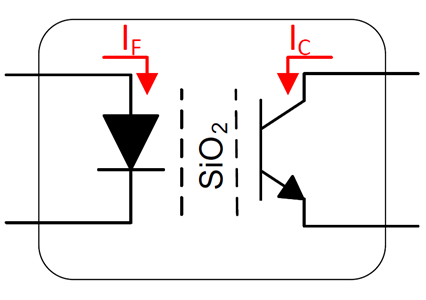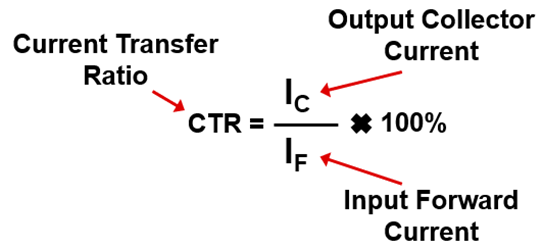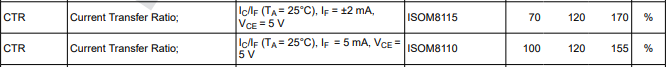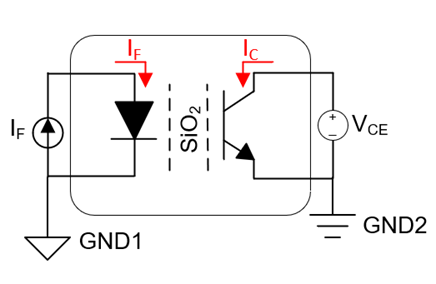If you have a related question, please click the "Ask a related question" button in the top right corner. The newly created question will be automatically linked to this question.

# [FAQ] What is Current Transfer Ratio (CTR)?

FAQ: Isolation >> Opto-Emulators >> Current FAQ

What is Current Transfer Ratio (CTR)?

• What is Current Transfer Ratio (CTR)?

Current Transfer Ratio (CTR) is a ratio of the current to the output collector input forward current and is represented in a percentage.

CTR is mainly characterized in analog opto-emulator or optocoupler devices.The input forward current (IF) is the current going into the diode while the output collector current (IC) is the current flowing into the output transistor.

• For CTR > 100%, the IC will be greater than the IF.
• For CTR < 100%, the IC will be less than the IF.

How is it represented in datasheets?How is CTR measured from a device?

The diagram below shows the test circuit which is designed to measure the CTR for an analog opto-emulator.By applying the input forward current and applying a voltage to the collector-emitter, the output collector current can be measured.